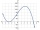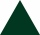# Arc and segment

Calculate the length of circular arc l, area of the circular arc S1 and area of circular segment S2. Radius of the circle is 33 and corresponding angle is.

Result

l =  29.6
S1 =  488.7
S2 =  63

#### Solution:Leave us a comment of this math problem and its solution (i.e. if it is still somewhat unclear...):Be the first to comment!#### Following knowledge from mathematics are needed to solve this word math problem:

Most natural application of trigonometry and trigonometric functions is a calculation of the triangles. Common and less common calculations of different types of triangles offers our triangle calculator. Word trigonometry comes from Greek and literally means triangle calculation.

## Next similar math problems:

1. ConeCircular cone of height 15 cm and volume 5699 cm3 is at one-third of the height (measured from the bottom) cut by a plane parallel to the base. Calculate the radius and circumference of the circular cut.
2. Right ΔA right triangle has the length of one leg 7 cm and length of the hypotenuse 25 cm. Calculate the height of the triangle.
3. TerezaThe cube has area of base 256 mm2. Calculate the edge length, volume and area of its surface.
4. Right triangleLegs of the right triangle are in the ratio a:b = 2:8. The hypotenuse has a length of 87 cm. Calculate the perimeter and area of the triangle.Calculate the radius of the circle whose length is 107 cm larger than its diameter
6. Square diagonalCalculate length of the square diagonal if the perimeter is 476 cm.
7. IS trapezoidCalculate the length of diagonal u and height v of isosceles trapezoid ABCD, whose bases have lengths a = |AB| = 37 cm, c = |CD| = 29 cm and legs b = d = |BC| = |AD| = 28 cm.
8. Cone A2VSurface of cone in the plane is a circular arc with central angle of 126° and area 415 cm2. Calculate the volume of a cone.
9. Reciprocal valueHow do I calculate a number x that is 9 greater than its reciprocal (1/x)?
10. Cube in a sphereThe cube is inscribed in a sphere with volume 9067 cm3. Determine the length of the edges of a cube.
11. MO SK/CZ Z9–I–3John had the ball that rolled into the pool and it swam in the water. Its highest point was 2 cm above the surface. Diameter of circle that marked the water level on the surface of the ball was 8 cm. Determine the diameter of John ball.
12. The tractorThe tractor sows an average of 1.5 ha per hour. In how many hours does it sows a rectangular trapezoid field with the bases of 635m and 554m and a longer arm 207m?
13. Rectangular cuboidThe rectangular cuboid has a surface area 5334 cm2, its dimensions are in the ratio 2:4:5. Find the volume of this rectangular cuboid.
14. An equilateral triangleThe perimeter of an equilateral triangle is 33cm. How long is each side?
15. Depth anglesAt the top of the mountain stands a castle, which has a tower 30 meters high. We see the crossroad in the valley from the top of the tower and heel at depth angles of 32° 50 'and 30° 10'. How high is the top of the mountain above the crossroad
16. TheaterThe theater has in each row with 19 seats. Ticket to the first 10 rows is for 30 USD. In next rows is for 15 USD. The theater was completely sold out. Revenue was 12255 USD. How many rows are in the theater?
17. Sea waterMixing 62 kg of sea water with 84 kg rainwater is created water containing 3.1% salt. How many percent sea water contains salt?